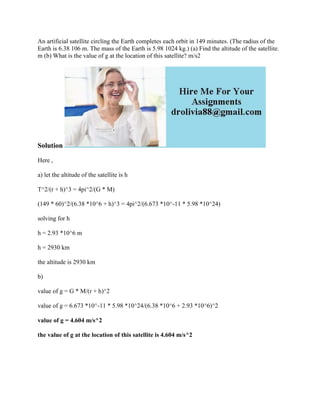Successfully reported this slideshow.

# An artificial satellite circling the Earth completes each orbit in 149.docx×

# An artificial satellite circling the Earth completes each orbit in 149.docx

An artificial satellite circling the Earth completes each orbit in 149 minutes. (The radius of the Earth is 6.38 106 m. The mass of the Earth is 5.98 1024 kg.) (a) Find the altitude of the satellite. m (b) What is the value of g at the location of this satellite? m/s2
Solution
Here ,
a) let the altitude of the satellite is h
T^2/(r + h)^3 = 4pi^2/(G * M)
(149 * 60)^2/(6.38 *10^6 + h)^3 = 4pi^2/(6.673 *10^-11 * 5.98 *10^24)
solving for h
h = 2.93 *10^6 m
h = 2930 km
the altitude is 2930 km
b)
value of g = G * M/(r + h)^2
value of g = 6.673 *10^-11 * 5.98 *10^24/(6.38 *10^6 + 2.93 *10^6)^2
value of g = 4.604 m/s^2
the value of g at the location of this satellite is 4.604 m/s^2
.

An artificial satellite circling the Earth completes each orbit in 149 minutes. (The radius of the Earth is 6.38 106 m. The mass of the Earth is 5.98 1024 kg.) (a) Find the altitude of the satellite. m (b) What is the value of g at the location of this satellite? m/s2
Solution
Here ,
a) let the altitude of the satellite is h
T^2/(r + h)^3 = 4pi^2/(G * M)
(149 * 60)^2/(6.38 *10^6 + h)^3 = 4pi^2/(6.673 *10^-11 * 5.98 *10^24)
solving for h
h = 2.93 *10^6 m
h = 2930 km
the altitude is 2930 km
b)
value of g = G * M/(r + h)^2
value of g = 6.673 *10^-11 * 5.98 *10^24/(6.38 *10^6 + 2.93 *10^6)^2
value of g = 4.604 m/s^2
the value of g at the location of this satellite is 4.604 m/s^2
.